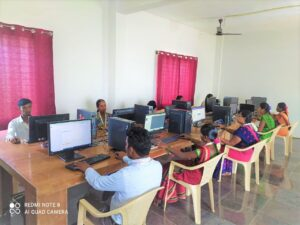Interdisciplinary Research Society

Data Analytics Lab

Data Analytics LabMatlab Software

Minitab Software

R Software

Python Software

SPSS Software

MS Excel

Following  Data Analysis was performed in our Lab

Making data visualizations; Create and Edit Graphs; Charts, and Plots,
Independent Samples T-test; Paired Samples T-test; Chi-Square Test; Normality Test; ANOVA,
Computing frequencies and correlations; Measures of Central Tendency and Dispersion; Pearson Correlation; Spearman Ranked Order Correlation; Kendall's Tau B Correlation; Point Bi-Serial Correlation,
Linear Regression, Multiple Regression,
Analyzing data with Pivot tables; What-if analysis?; Forecasting; Sampling;  Fourier analysis,
MCDM methods: Weight allocation methods; Mean weight method; Standard deviation method; Entropy method; AHP method; Critic Method and Best Worst method; VIKOR Method; ELECTRE Method; Promethee Method; Additive Ratio Assessment (ARAS); Preference Selection Index (PSI); Complex Proportional Assessment (COPRAS); Weighted Aggregated Sum Product Assessment (WASPAS); Evaluation Based on Distance from Average Solution (EDAS); Decision Making Trial and Evaluation Laboratory method (DEMATEL); Fuzzy-ARAS
Structural Equation Model: Model Identification; Testing of Model Fitness; Multi-Group Analysis; Multi-Collinearity Test; Multiple Model Analysis; Confirmatory Factor Analysis; Path Analysis
Optimization: Genetic Algorithm; Multi Genetic Algorithm; Gradient-based Algorithm; PSO Optimisation Algorithm; Jaya Algorithm; Harmony Search Algorithm; Teaching Learning Based Optimization